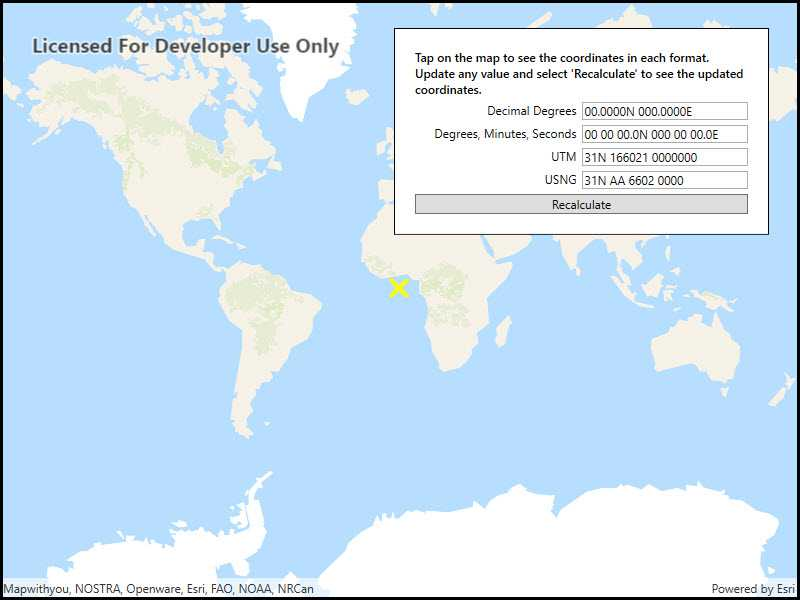# Format coordinates

View inWPFUWPFormsiOSAndroid
View on GitHub
Sample viewer app

Format coordinates in a variety of common notations.## Use case

The coordinate formatter can format a map location in WGS84 in a number of common coordinate notations. Parsing one of these formats to a location is also supported. Formats include decimal degrees; degrees, minutes, seconds; Universal Transverse Mercator (UTM), and United States National Grid (USNG).

## How to use the sample

Click on the map to see a callout with the clicked location's coordinate formatted in 4 different ways. You can also put a coordinate string in any of these formats in the text field. Hit Enter and the coordinate string will be parsed to a map location which the callout will move to.

## How it works

1. Get or create a `MapPoint` with a spatial reference.
2. Use one of the static "to" methods on `CoordinateFormatter` such as `CoordinateFormatter.ToLatitudeLongitude(point, CoordinateFormatter.LatitudeLongitudeFormat.DecimalDegrees, 4)` to get the formatted string.
3. To go from a formatted string to a `Point`, use one of the "from" static methods like `CoordinateFormatter.FromUtm(coordinateString, map.SpatialReference, CoordinateFormatter.UtmConversionMode.NorthSouthIndicators)`.

## Relevant API

• CoordinateFormatter
• CoordinateFormatter.LatitudeLongitudeFormat
• CoordinateFormatter.UtmConversionMode

## Tags

convert, coordinate, decimal degrees, degree minutes seconds, format, latitude, longitude, USNG, UTM

## Sample Code

FormatCoordinates.xamlFormatCoordinates.xaml.cs

``````﻿<UserControl x:Class="ArcGISRuntime.WPF.Samples.FormatCoordinates.FormatCoordinates"
xmlns="http://schemas.microsoft.com/winfx/2006/xaml/presentation"
xmlns:x="http://schemas.microsoft.com/winfx/2006/xaml"
xmlns:esri="http://schemas.esri.com/arcgis/runtime/2013">
<UserControl.Resources>
<Style TargetType="TextBlock">
<Setter Property="Margin" Value="0,5,5,0" />
</Style>
<Style TargetType="TextBox">
<Setter Property="Margin" Value="0,5,0,0" />
</Style>
</UserControl.Resources>
<Grid>
<esri:MapView x:Name="MyMapView" />
<Border Style="{StaticResource BorderStyle}">
<Grid>
<Grid.RowDefinitions>
<RowDefinition Height="Auto" />
<RowDefinition Height="Auto" />
<RowDefinition Height="Auto" />
<RowDefinition Height="Auto" />
<RowDefinition Height="Auto" />
<RowDefinition Height="Auto" />
</Grid.RowDefinitions>
<Grid.ColumnDefinitions>
<ColumnDefinition Width="*" />
<ColumnDefinition Width="*" />
</Grid.ColumnDefinitions>
<TextBlock
Text="Tap on the map to see the coordinates in each format. Update any value and select 'Recalculate' to see the updated coordinates."
Grid.Row="0" Grid.Column="0" Grid.ColumnSpan="2"
Margin="0,0,0,0"
TextWrapping="Wrap" FontWeight="SemiBold" />
<TextBlock Text="Decimal Degrees"
Grid.Row="1" Grid.Column="0"
HorizontalAlignment="Right" />
<TextBox x:Name="DecimalDegreesTextField"
Grid.Row="1" Grid.Column="1"
Tag="Decimal Degrees" />
<TextBlock Text="Degrees, Minutes, Seconds"
Grid.Row="2" Grid.Column="0"
HorizontalAlignment="Right" />
<TextBox x:Name="DmsTextField"
Grid.Row="2" Grid.Column="1"
Tag="Degrees, Minutes, Seconds" />
<TextBlock Text="UTM"
Grid.Row="3" Grid.Column="0"
HorizontalAlignment="Right" />
<TextBox x:Name="UtmTextField"
Grid.Row="3" Grid.Column="1"
Tag="UTM" />
<TextBlock Text="USNG"
Grid.Row="4" Grid.Column="0"
HorizontalAlignment="Right" />
<TextBox x:Name="UsngTextField"
Grid.Row="4" Grid.Column="1"
Tag="USNG" />
<Button Content="Recalculate"
Grid.Row="5" Grid.Column="0" Grid.ColumnSpan="2"
Margin="0,5,0,0"
Click="RecalculateFields" />
</Grid>
</Border>
</Grid>
</UserControl>
``````